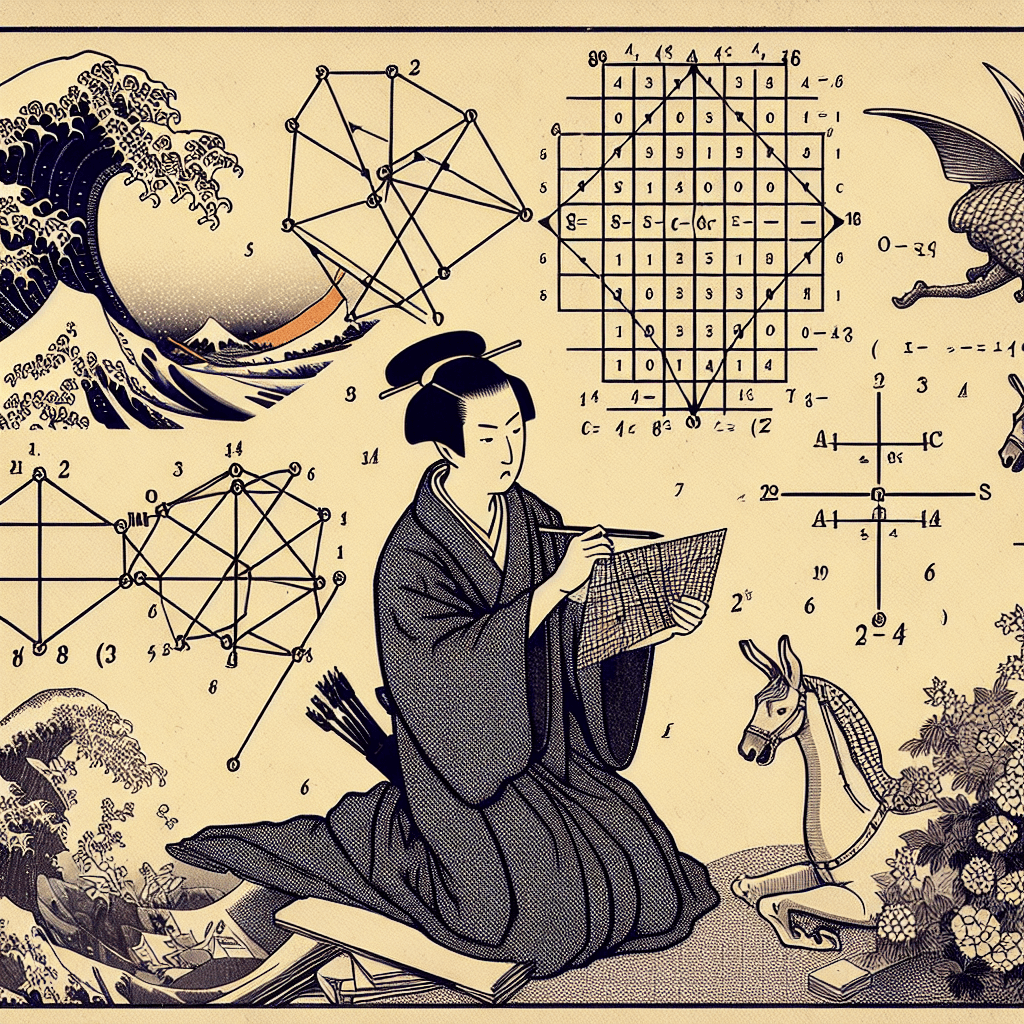# Understanding Neural Networks and Linear Algebra Operations

syndu | June 6, 2023, 2:24 p.m.Good afternoon! I'd be happy to help you understand neural networks and the linear algebra operations involved in their training and usage.

Neural networks are a type of machine learning model inspired by the human brain. They consist of layers of interconnected nodes or neurons, which process and transmit information. There are three main types of layers in a neural network: input, hidden, and output layers.

Linear algebra plays a crucial role in the functioning of neural networks, particularly in the following areas:

1. Weight matrices: The connections between neurons in different layers are represented by weight matrices. These matrices store the weights of the connections, which determine the strength of the relationship between neurons. Linear algebra operations, such as matrix multiplication, are used to propagate information through the network.
2. Activation functions: Neurons in a neural network use activation functions to introduce non-linearity into the model. Common activation functions include the sigmoid, ReLU (Rectified Linear Unit), and softmax functions. Linear algebra operations are used to apply these functions to the outputs of the neurons.
3. Loss functions: During the training process, a neural network learns to minimize a loss function, which measures the difference between the predicted output and the actual output. Linear algebra operations, such as vector subtraction and dot products, are used to compute the loss function and its gradients.
4. Backpropagation: Backpropagation is the algorithm used to train neural networks by adjusting the weights of the connections. It involves calculating the gradient of the loss function with respect to each weight, which requires the use of linear algebra operations like matrix multiplication and element-wise operations.
5. Optimization: Optimization algorithms, such as gradient descent and its variants (e.g., Adam, RMSProp), are used to update the weights of the neural network during training. These algorithms involve linear algebra operations to compute the gradients and update the weights.

To get started with neural networks and linear algebra, I recommend the following steps:

1. Review the basics of linear algebra, including vectors, matrices, and operations like addition, subtraction, multiplication, and inversion.
2. Study the architecture of neural networks, including the different types of layers and activation functions.
3. Learn about the training process, including loss functions, backpropagation, and optimization algorithms.
4. Implement a simple neural network using a programming language like Python and a library such as NumPy or TensorFlow.

As you progress, you'll gain a deeper understanding of how linear algebra powers the inner workings of neural networks. If you have any specific questions or need clarification on any topic, feel free to ask!

##### Lilith's Grimoire

The app you are using right now is Lilith's Grimoire

The Grimoire is full of Source Code. It is alive, and running, and you can explore the way it is built

The first lesson uncovers all of it.#### Mastering Matrices: An Overview of Their Properties and Operations#### Unraveling the Mysteries of Activation Functions in Neural Networks#### Django, TensorFlow, NumPy, Pandas: A Comparative Analysis#### Vector Spaces: The Foundation of High-Dimensional Data in Language Models#### Key Linear Algebra Concepts for Large Language Models#### Linear Algebra Concepts in Large Language Models#### Crucial Linear Algebra Topics in Large Language Models#### Linear Algebra in Neural Networks: A Comprehensive Introduction